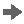Show TOC

###Variance CategoriesReport that you can use with product drilldown to analyze the individual variance categories for the following application components:

• Product Cost by Order

• Product Cost by Period

The reporting time frame can be cumulative or limited.

This report makes it possible for you to analyze the results of the variance calculation. The results of variance calculation are different depending on the target cost version specified for the report.

You can compare the following costs:

• Target costs that have been calculated on the basis of standard costs against actual costs (without work in process and scrap variances).

• Target costs that have been calculated on the basis of a preliminary cost estimate of an order (product cost collector or manufacturing order) against actual costs (without work in process and scrap variances).

• Target costs that have been calculated on the basis of an alternative material cost estimate (for example, modified standard cost estimate, current cost estimate) against net actual costs (without work in process and scrap variances).

• Target costs calculated on the basis of standard costs against planned costs of manufacturing orders.

#### Integration

##### Variance Categories

The following variance categories are shown based on key figures and/or original cost elements:

• Scrap variance

• Input price variance

• Input quantity variance

• Resource-usage variance

• Remaining input variance

• Mixed price variance

• Output price variance

• Lot size variance

• Remaining variance

• Object information

• Reporting time frame

#### Prerequisites

Make sure that the variances have been calculated for the specific target cost version in the relevant reporting time frame.

Reports for summarized analysis require that a data collection run be executed beforehand.Recommendation

This report is intended for more detailed analysis of target / actual / production variances. You can normally navigate from product drilldowns to the variance categories report.

End of the recommendation.

In the documentation for Cost Object Controlling:

Basic information on variance calculation can be found in:

Variance Calculation

##### Detailed information on the individual variance categories can be found in the following:

Variance Categories

Scrap Variance

Information on the target cost versions and their resulting comparison values can be found in the following:

Types of Variance Calculation

Target Cost Versions in Variance Calculation

Calculation of Target Costs

Calculation of Control Costs